# Servo Brightness Gauge

An R/C servo can be used to make a gauge to measure the intensity of light.

BeginnerProtip2 hours2,015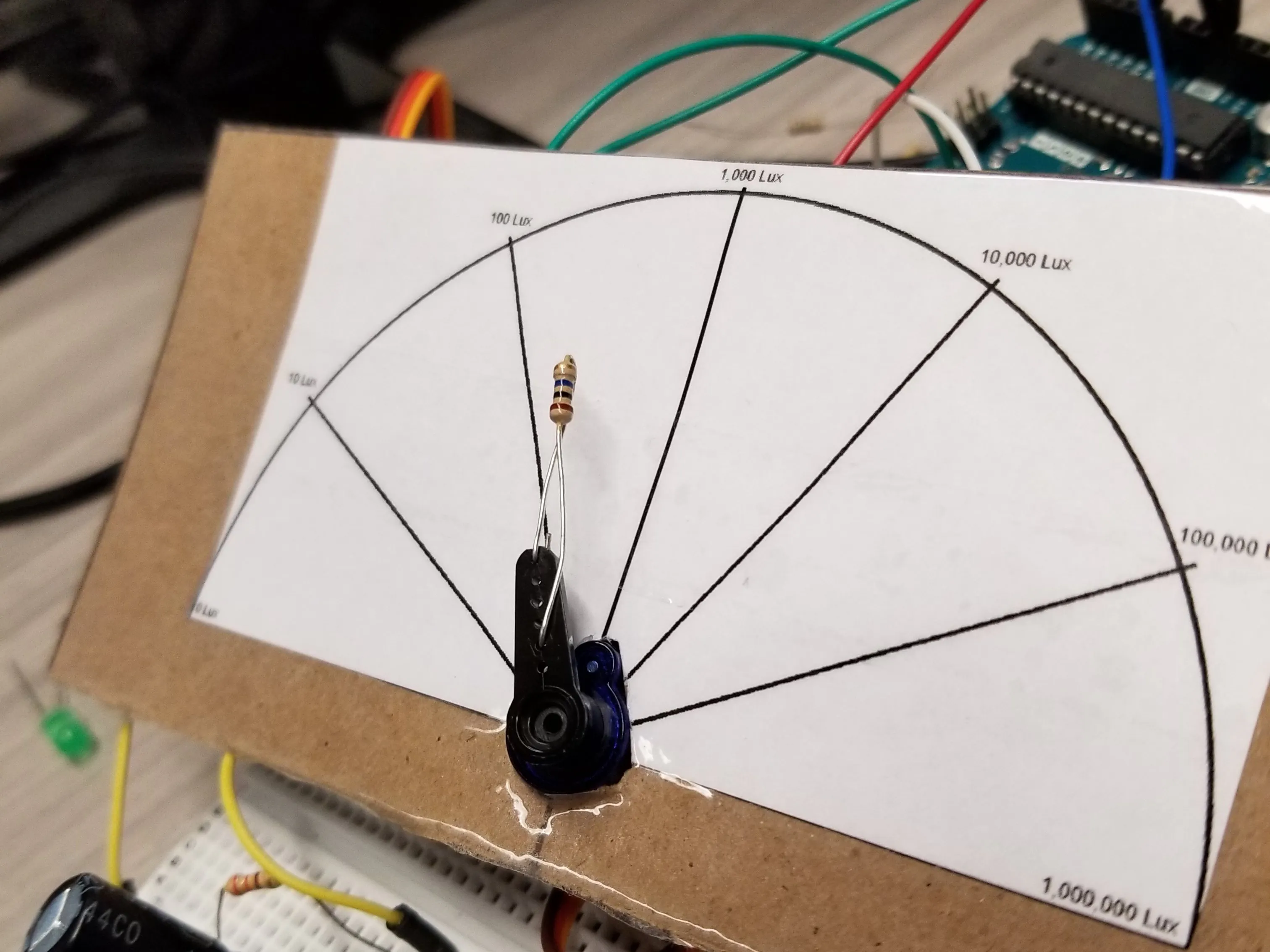## Things used in this project

### Hardware components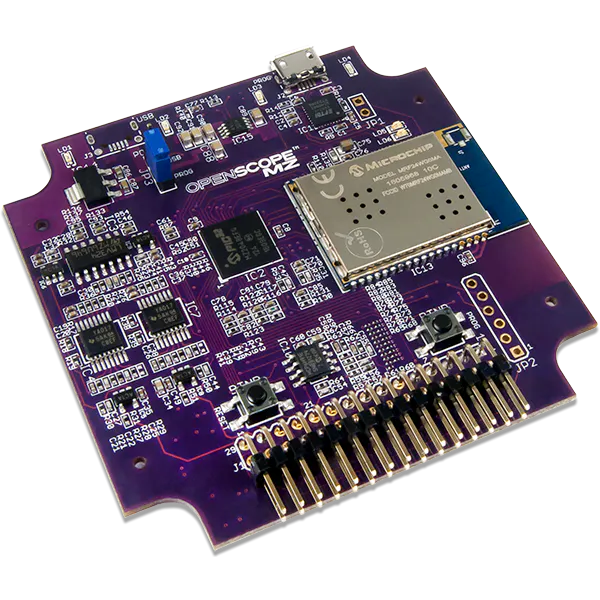Digilent OpenScope MZ
×1Arduino UNO
×1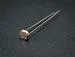Photo resistor
×1
 Servos (Tower Pro MG996R) This project uses a 180° servo, but beyond that choose whatever model you want.
×1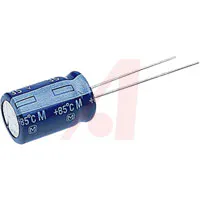Capacitor 1000 µF
×2

### Software apps and online services

 Digilent WaveForms LiveArduino IDE

## Schematics

### Servo Lux Gauge

The image I created to make the brightness gauge.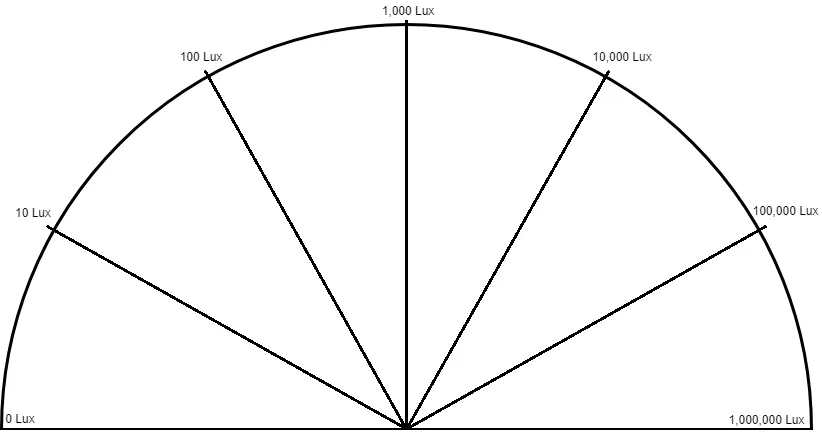## Code

### Servo Brightness Gauge

Arduino
This code measures a photoresistor voltage, converts it to lux, and stores the running average of the value. It then moves the servo to reflect the value on the gauge.
```#include <Servo.h>
#include <math.h>

//variables to keep a running average of the samples
float total;
float avg;

//number of samples to have in the running average
const int numSmpl = 10;

// create a servo object
Servo servo;

int photoVal = A0;

/*
equations to convert voltage to lux values:
0-.534V:
lux = 1286.47506604*pow(voltage,2) + 178.51342186*voltage - 1.70982528

>0.534-2.9V:
lux = 197.90983722e^(1.62826391*voltage)

>2.9 - 4.1V:
lux = -3785.88415527*voltage^6 + 85678.15521655*voltage^5 - 604387.00259741*voltagets^4 + 1410432.63046882*voltage^3 + 1529553.55029751*voltage^2 - 11122170.790418*voltage + 12440209.3288285

>4.1V:
lux = 23.9858393e^(2.28356737*voltage)

equation to convert lux value to degrees on servo:
y = 13.0288344570976*ln(voltage) - 0.0000000000000560135

*/

void setup() {
// link the servo to pin 9, setting the pulse width limits
servo.attach(9, 544, 2660);

//set the pins as inputs and outputs
pinMode(photoVal, INPUT);
analogWrite(LED,1);
Serial.begin(9600);
//take an initial sampling of measurements
total = 0;
for(int i = 0; i < numSmpl; i++){
delay(1);
}
//average the samples out
avg = total/numSmpl;
}

void loop() {
//position the servo based on the running average
setServo(sampleBuffer());

//give the servo time to move
delay(15);
}

//performs a reading of the photoresistor and converts the voltage to lux
//store the potentiometer position as a float

//calculate analog voltage
float voltage = 5*level/1024;

//make sure the voltage isn't outside the acceptable range
if(voltage < 0){
voltage = 0;
}
if(voltage > 5){
voltage = 5;
}
//initialize a lux value
float lux = 0;

//based on the voltage reading, select the equation that will best convert it to a lux value
if(voltage <= 0.534){
lux = 1286.47506604*pow(voltage,2) + 178.51342186*voltage - 1.70982528;
}

else if(voltage <= 2.9){
lux = 197.90983722*exp(1.62826391*voltage);
}

else if(voltage <= 4.1){
lux = -3785.88415527*pow(voltage,6) + 85678.15521655*pow(voltage,5) - 604387.00259741*pow(voltage,4) + 1410432.63046882*pow(voltage,3) + 1529553.55029751*pow(voltage,2) - 11122170.790418*voltage + 12440209.3288285;
}

else{
lux = 23.9858393*exp(2.28356737*voltage);
}
if(lux < 0 ){
lux = 0;
}
return lux;
}

//performs a modified running average on the lux measurements
float sampleBuffer(){

//subtract previous average
total -= avg;

//calculate new average
avg = total/numSmpl;
return avg;
}

//moves the servo to a new position
void setServo(float lux){
//map lux values to 180-degree scale
int pos = round(13.0288344570976*log(lux) - 0.0000000000000560135);
if(pos < 0){
pos = 0;
}
//position value is reversed to make gauge indicate from left to right
servo.write(180 - pos);
}
```

## Credits

### Boris Leonov

10 projects • 22 followers
Electrical engineering student in the Seattle area. Electrical and mechanical DIY enthusiast. Fixer of things, large and small.

### Sam Kristoff

35 projects • 49 followers
Director of Engineering at Digilent.

### Arthur Brown

14 projects • 28 followers
Applications engineer and digital logic geek